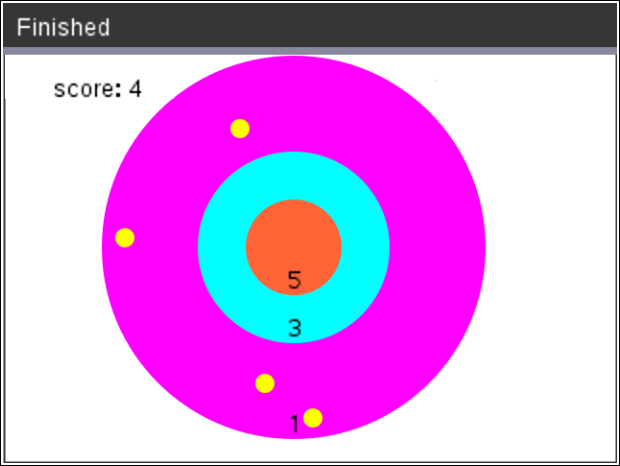# Activities

•• ##### Subject Area

• Math: Explorations with Coding: Coding with Python

6-8

• ##### Device
•TI-Nspire™ CX II
• ##### Software

TI-Nspire™ CX

5.4

## Integer Darts#### Activity Overview

In this coding activity, students will create an integer dart game using Python and mathematics.

#### Objectives

• Use variables to store values
• Use the randint() function to generate integers
• Use the print() function to display
• Use a while loop to repeat code.
• Use an if..elif statements to make decisions
• Add and subtract integers
• Multiply and divide integers.
• Use Pythagorean Theorem to find distance between two points (May be omitted. To skip, give students Template 2 instead of Template 1, and stop coding after step 13.)

#### About the Lesson

In this project, you will create an integer dart game.  Part of the code has already been written in the “Integer Darts Template.tns” file.  You will write the code to generate integer addition, subtraction, multiplication and division problems. You will also write the code to determine the score.  Each correctly answered integer question will earn a dart.

Note: This lesson requires the use of TI-Nspire™ CX II technology with OS 5.2 and above.